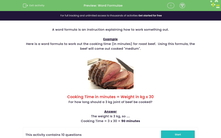# Word Formulae

In this worksheet, students use simple word formulae to work out the answers.Key stage:  KS 2

Curriculum topic:   Number: Multiplication and Division

Curriculum subtopic:   Solve Multiplication/Division Problems

Difficulty level:#### Worksheet Overview

A word formula is an instruction explaining how to work something out.

Example

Here is a word formula to work out the cooking time (in minutes) for roast beef.  Using this formula, the beef will come out cooked "medium".Cooking Time in minutes = Weight in kg x 30

For how long should a 3 kg joint of beef be cooked?